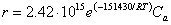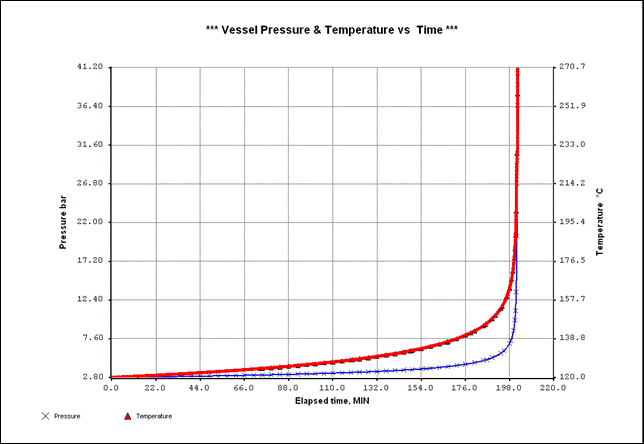A FUGITIVE REACTION

View:               Process Flow Diagram

# Problem Description

The purpose of this example is to show the simulation of adiabatic thermal decomposition of di-tert-butyl peroxide (DTBP) with the aim of comparing results with data obtained from ‘Accelerating Rate Calorimeter’ (ARC) experiments.

DTBP is an industrially important organic peroxide widely used as an initiator for radical polymerization, a source for alkoxyl radicals, and a fuel combustion additive and used in reforming operations.

DTBP is usually stored in solution, most frequently used solvents are toluene and benzene.

The simulation describes the decomposition of a DTBP solution (20% weight) in toluene.

A large number of papers can be found about the kinetic rate and mechanism of the DTBP decomposition suggesting a number of possible decomposition products.

In this simulation a simple model is used.

The decomposition of 1 mole of DTBP produces 1 mole of ethane and 2 moles of acetone (dimethyl-ketone).

This is expressed, using the XPSIM keyword syntax as:

STOICH DTBP -1 / C2 1 / DMK 2

Reaction kinetic is modeled as a first order reaction in the DTBP concentration as:where Ca is the molar concentration of DTBP.

The exothermal heat of reaction is set to 280000 kJ/kmol (or 280 kJ/mol)

The calorimeter is modeled as a vessel, the DTBP solution is specified to occupy 70% of the volume of the calorimeter. The remaining part of the vessel is filled by air.

Initial conditions are 120°C at a pressure of 2.8 bar.

The diagram of the vessel temperature and pressure showing the decomposition is presented on Fig. 1.

Fig. 1Generated Keyword Input File

<XPSIM>  ...generated by XpsimWin v.1.07 ...

*

RUN ID=DTP CUSTOMER=STAFF PROBLEM='DTP DECOMPOSITION'

DESC DTP - DI TERT BUTYL PEROXIDE IN TOLUENE

DESC DECOMPOSITION

DESC TO BE USED TO INCLUDE A REACTION IN A DEPRESSURIZER

DIMENSION INPUT SI PRES=BAR

System Data

CHEMCOMP DTBP / TOLU / C2 / DMK / O2 / N2

THERMSET UID=M1

METHODS K=SRK FV=SRK FL=SRK HS=LK CP=LK D=LK IV=LK

Reaction Data

RXSET UID=R1

RX UID=DECO

DESC PEROXIDE DECOMPOSITION

STOICH DTBP -1 / C2 1 / DMK 2

KRATE BASIS=MOLARITY PHASE=LIQ PEF=2.42E15 AE=151430

POWLAW DTBP 1.0

PROPERTY PHASE=LIQ DH0=-280000

Flowsheet Data

STREAM=S1 TEMP=50 PRES=1 RATE(W)=1000 XBASIS=W

COMP DTBP:20 / TOLU:80 / C2:0 / DMK:0

STREAM=AIR TEMP=50 PRES=1 RATE(W)=100 XBASIS=V

COMP DTBP:0 / TOLU:0 / C2:0 / DMK:0 / O2:21 / N2:79

FLASH IN AIR S1 OUT F1CELL UID=F1

CALC ISO TEMP=120 PRES=2.8

DEPRESS IN F1CELL OUT FREL TREL FCONT IREL UID=DEPR1

VESSEL VOLUME=1 CHARGE(V)=0.7 RATIO FEED=LIQ

CALC MODEL=1 PHASE=MIX PRELIEF=41 PFINAL=7 TFINAL(MIN)=300 +

TDEPRES(MIN)=1 TSTEP(SEC)=10 NOSTOP

REAC SET=R1

PSV MODEL=2 CONST=100.

<DISABLE>

HEAT MODEL=1 AREA=0.1

</DISABLE>

PRINT PLOT TINT(MIN)=5 TRACE

PRODUCT IRELIEF=IREL FRELIEF=FREL FCONTENT=FCONT TRELIEF=TREL

Recycle Data

SOLUTION NOCONV=CONTINUE

END